# Chapter 3: Pair of Linear Equations in Two Variables Exercise – 3.4

### Question: 1

Solve the system of equations:

x + 2y + 1 = 0 and 2x – 3y – 12 = 0

### Solution:

x + 2y + 1 = 0 …. (i)

2x - 3y - 12 = 0 …….. (ii)

Here a1 = 1, b1 = 2, c1 = 1

a2 = 2, b2 = -3, c2 = – 12

By cross multiplication method,Now,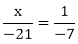x = 3

And,= y = - 2

The solution of the given system of equation is 3 and – 2 respectively.

### Question: 2

Solve the system of equations:

3x + 2y + 25 = 0, 2x + y + 10 = 0

### Solution:

3x + 2y + 25 = 0 …. (i)

2x + y + 10 = 0 ….. (ii)

Here a1 = 3, b1 = 2, c1 = 25

a2 = 2, b2 = 1, c2 = 10

By cross multiplication method,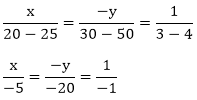Now,x = 5

And,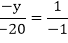y = – 20

The solution of the given system of equation is 5 and -20 respectively.

### Question: 3

Solve the system of equations:

2x + y = 35, 3x + 4y = 65

### Solution:

2x + y = 35 …. (i)

3x + 4y = 65 ….. (ii)

Here a1 = 2, b1 = 1, c1 = 35

a2 = 3, b2 = 4, c2 = 65

By cross multiplication method,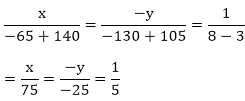Now,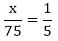x = 15

And,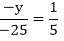y = 5

The solution of the given system of equation is 15 and 5 respectively.

### Question: 4

Solve the system of equations:

2x – y – 6 = 0, x – y – 2 = 0

### Solution:

2x - y = 6 …. (i)

x - y = 2 ….. (ii)

Here a1 = 2, b1 = -1, c1 = 6

2= 1, b2 = -1, c2 = 2

By cross multiplication method,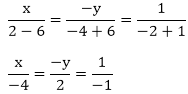Now,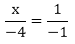x = 4

And,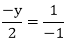y = 2

The solution of the given system of equation is 4 and 2 respectively

### Question: 5

Solve the system of equations: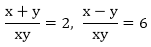### Solution: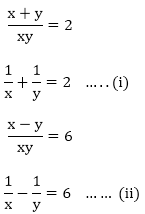Taking 1/x = u

Taking 1/y = v

u + v = 2 …… (iii)

u - v = 6 ….  (iv)

By cross multiplication method,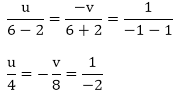Now,

u/4 = 1/-2

u = - 2

And,

- v/ – 8 = 1/ – 2

v = – 4

1/u = x

X = – 1/2

y = –1/4

The solution of the given system of equation is -1/2 and -1/4 respectively.

### Question: 6

Solve the system of equations:

ax + by = a – b, bx - ay = a + b

### Solution:

ax + by = a - b …. (i)

bx - ay = a + b ….. (ii)

Here a1 = a, b1 = b, c1 = a - b

a2 = b, b2 = -a, c2 = a + b

By cross multiplication method,Now,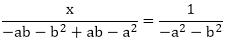x = 1

And,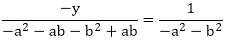y = – 1

The solution of the given system of equation is 1 and -1 respectively.

### Question: 7

Solve the system of equations:

x + ay - b = 0, ax - by - c = 0

### Solution:

x + ay - b = 0 ….. (i)

ax - by - c = 0 ……. (ii)

Here a1 = 1, b1 = a, c1 = - b

a2 = a, b2 = - b , c2 = - c

By cross multiplication method,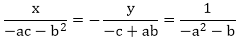Now,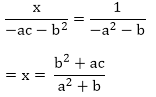And,The solution of the given system of equation isrespectively.

### Question: 8

Solve the system of equations:

ax + by = a2

bx + ay = b2

### Solution:

ax + by = a2 …. (i)

bx + ay = b2 ….. (ii)

Here a1 = a, b1 = b, c1 = a2

a2 = b, b2 = a, c2 = b2

By cross multiplication method,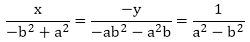Now,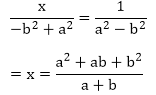And,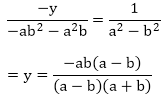The solution of the given system of equation isrespectively.

### Question: 9

Solve the system of equations: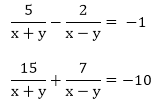### Solution: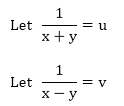The given system of equations are:

5u - 2v = -1

15u + 7v = -10

Here a1 = 5, b1 = – 2, c1 = 1

a2 = 15, b2 = 7, c2 = 10

By cross multiplication method,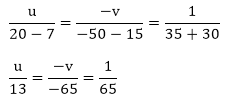Now,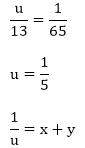x + y = 5 …. (i)

And,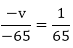v = 1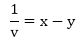x - y =  1 …… (ii)

2x = 6

x = 3

Putting the value of x in equation (i)

3 + y = 5

y = 2

The solution of the given system of equation is 3 and 2 respectively.

### Question: 10

Solve the system of equations:### Solution:

Let 1/x = u

Let 1/y = v

The given system of equations becomes:

2u + 3v = 13 …… (i)

5u - 4v = – 2 …. (ii)

By cross multiplication method,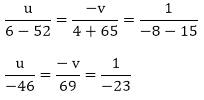Now,u = 2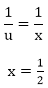And,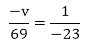v = 3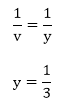The solutions of the given system of equations are 1/2 and 1/3 respectively.

### Question: 11

Solve the system of equations: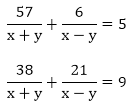### Solution:The given system of equations are:

57u + 6v = 5

38u + 21v = 9

Here a1 = 57, b1 = 6, c1 = – 5

a2 = 38, b2 = 21, c2 = – 9

By cross multiplication method,Now,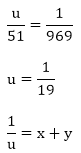x + y = 19 ….. (i)

And,x - y = 3 … (ii)

2x = 22

x = 11

Putting the value of x in equation (i)

11 + y = 19

y = 8

The solution of the given system of equation is 11 and 8 respectively.

### Question: 12

Solve the system of equations: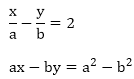### Solution:a2 = a, b2 = – b, c2 = b2 - a2

By cross multiplication methodx = a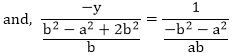y = b

Hence the solution of the given system of equation are a and b respectively.

### Question: 13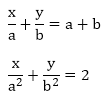### Solution: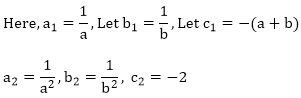By cross multiplication method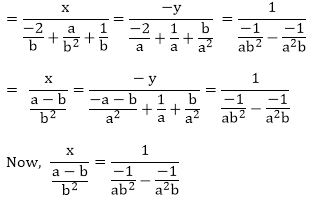x = a2y = b2

The solution of the given system of equation are a2  and brespectively.

### Question: 14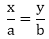ax + by = a2 + b2

### Solution: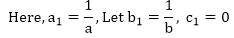Here, a1 = a, b2 = b, Let c1 = – (a2 + b2)

By cross multiplication method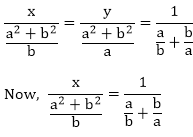x = a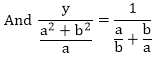y = b

The solution of the given system of equations are a and b respectively.

### Question: 15

2ax + 3by = a + 2b

3ax + 2by = 2a + b

### Solution:

The given system of equation is

2ax + 3by = a + 2b …… (i)

3ax + 2by = 2a + b ….. (ii)

Here a1 = 2a, b1 = 3b, c1 = – (a + 2b)

a2 = 3a, b2 = 2b, c2 = – (2a + b)

By cross multiplication method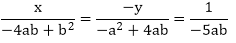Now,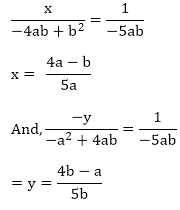The solutions of the system of equations are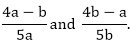respectively.

5ax + 6by = 28

3ax + 4by = 18

### Solution:

The systems of equations are:

5ax + 6by = 28 …. (i)

3ax + 4by = 18  …. (ii)

Here a1 = 5a, b1 = 6b, c1 = – (28)

a2 = 3a, b2 = 4b, c2 = – (18)

By cross multiplication method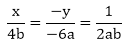Now,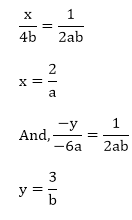The solution of the given system of equation is 2/a and 3/b.

### Question: 17

(a + 2b)x + (2a - b)y = 2

(a – 2b)x + (2a + b)y = 3

### Solution:

The given system of equations are:

(a + 2b)x + (2a - b)y = 2 ……. (i)

(a - 2b)x + (2a + b)y = 3 ….. (ii)

Here a1 = a + 2b, b1 = 2a - b, c1 = - (2)

a2 = a - 2b, b2 = 2a + b, c2 = - (3)

By cross multiplication method: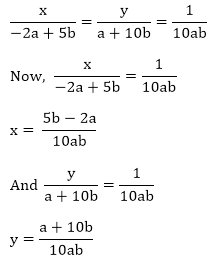The solution of the system of equations are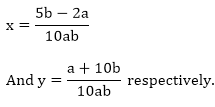### Question: 18

Solve the system of equations: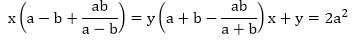### Solution:

The given systems of equations are:From equation (i)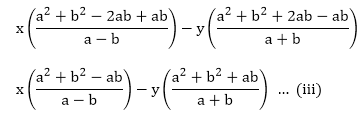From equation (ii)

x + y - 2a2 = 0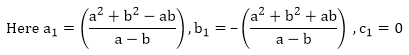a2 = 1, b2 = 1, c2 = – 2a2

By cross multiplication method: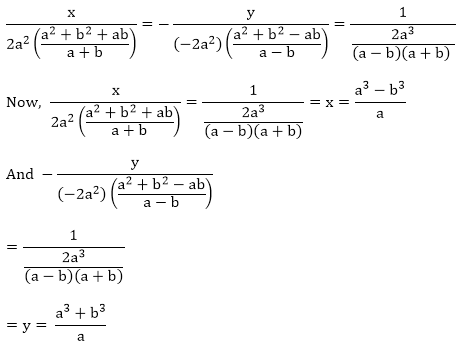The solutions of the given system of equations are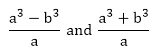respectively.

### Question: 19

Solve the system of equations: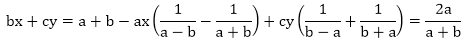### Solution:

The system of equation is given by:

bx + cy = a + b ……. (i)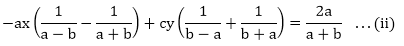From equation (i)

bx + cy - (a + b) = 0

From equation (ii)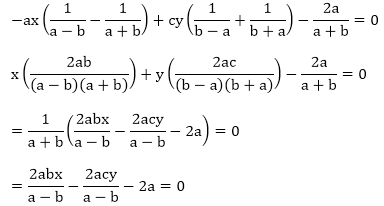2abx - 2acy - 2a(a - b) = 0 …. (iv)

By cross multiplicationx = a/b

And,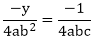y = b/c

The solution of the system of equations are a/b and b/c.

### Question: 20

Solve the system of equations:

(a - b)x +(a + b)y = 2a2 - 2b2(a + b)(x + y) = 4ab

### Solution:

The given system of equations are:

(a - b)x + (a + b)y = 2a2 - 2b….. (i)

(a + b)(x + y) = 4ab      …… (ii)

From equation (i)

(a - b)x + (a + b)y - 2a2 - 2b= 0

= (a - b)x + (a + b)y - 2(a2 - b2) = 0

From equation (ii)

(a - b)x + (a - b)y - 4ab = 0

Here, a1 = a - b, b= a + b, c1 = - 2(a2 + b2)

Here, a2 = a + b, b= a + b, c2 = - 4ab

By cross multiplication method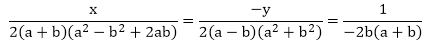Now,The solution of the system of equations are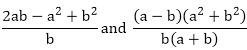respectively.

### Question: 21

Solve the system of equations:

a2x + b2y = c2

b2x + a2y = d2

### Solution:

The given system of equations are:

a2x + b2y = c….. (i)

b2x + a2y = d2 …… (ii)

Here, a1 = a2, b1 = b2, c1 = – c2

Here, a2 = b2, b= a2, c2 = – d2

By cross multiplication method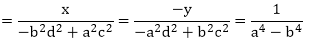Now,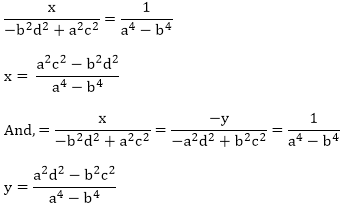The solution of the given system of equations arerespectively.

### Question: 22

Solve the system of equations:

2(ax - by + a + 4b = 0

2(bx + ay) + b - 4a = 0

### Solution:

The given system of equation may be written as:

2(ax - by + a + 4b = 0 ….. (i)

2(bx + ay) + b - 4a = 0 …. (ii)

Here, a1 = 2a, b= -2b, c1 = a + 4b

Here, a2 = 2b, b= 2a, c2 = b - 4a

By cross multiplication method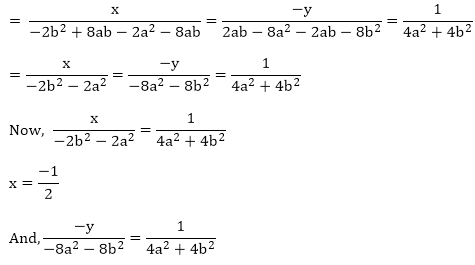y = 2

The solution of the given pair of equations are -1/2 and 2 respectively.

### Question: 23

Solve the system of equations:

6(ax + by) = 3a + 2b

6(bx - ay) = 3b - 2a

### Solution:

The systems of equations are

6(ax + by) = 3a + 2b …… (i)

6(bx - ay) = 3b - 2a ……. (ii)

From equation (i)

6ax + 6by - (3a + 2b) = 0  …… (iii)

From equation (ii)

6bx - 6ay - (3b - 2a) = 0 …… (iv)

Here, a1 = 6a, b= 6b, c1 = - (3a + 2b)

Here, a2 = 6b, b= -6a, c2 = - (3b - 2a)

By cross multiplication method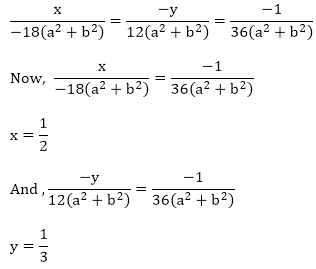The solution of the given pair of equations are 1/2 and 1/3 respectively.

### Question: 24

Solve the system of equations:### Solution:

The given systems of equations areTaking 1/x = u

Taking 1/y = v

The pair of equations becomes:

a2u - b2v = 0

a2bu + b2av - (a + b) = 0

Here, a1 = a2, b= -b2, c1 = 0

Here, a2 = a2b, b= b2a, c2 = – (a + b)

By cross multiplication method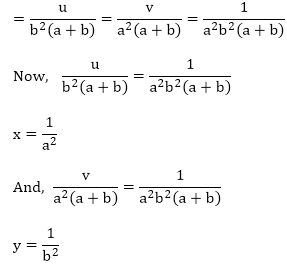The solution of the given pair of equations are 1/a2 and 1/b2 respectively.

### Question: 25

Solve the system of equations:

mx - my = m2 + n2

x + y = 2m

### Solution:

mx - my = m2 + n2 …… (i)

x + y = 2m …….. (ii)

Here, a1 = m, b= -n, c1 = - (m2 + n2)

Here, a2 = 1, b= 1, c2 = - (2m)

By cross multiplication methodx = m + ny = m - n

The solutions of the given pair of equations are m+n and m-n respectively.

### Question: 26

Solve the system of equations:ax - by = 2ab

### Solution:

The given pair of equations are: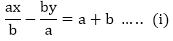ax - by = 2ab ….. (ii)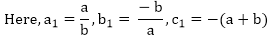Here, a2 = a, b= – b, c2 = - (2ab)

By cross multiplication methodx = by = – a

The solution of the given pair of equations are b and – a respectively.

### Question: 27

Solve the system of equations: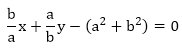x + y - 2ab = 0 ……. (ii)

### Solution: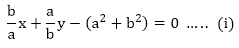x + y - 2ab = 0 ……. (ii)By cross multiplication method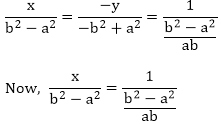x = aby = ab

The solutions of the given pair of equations are ab and ab respectively.